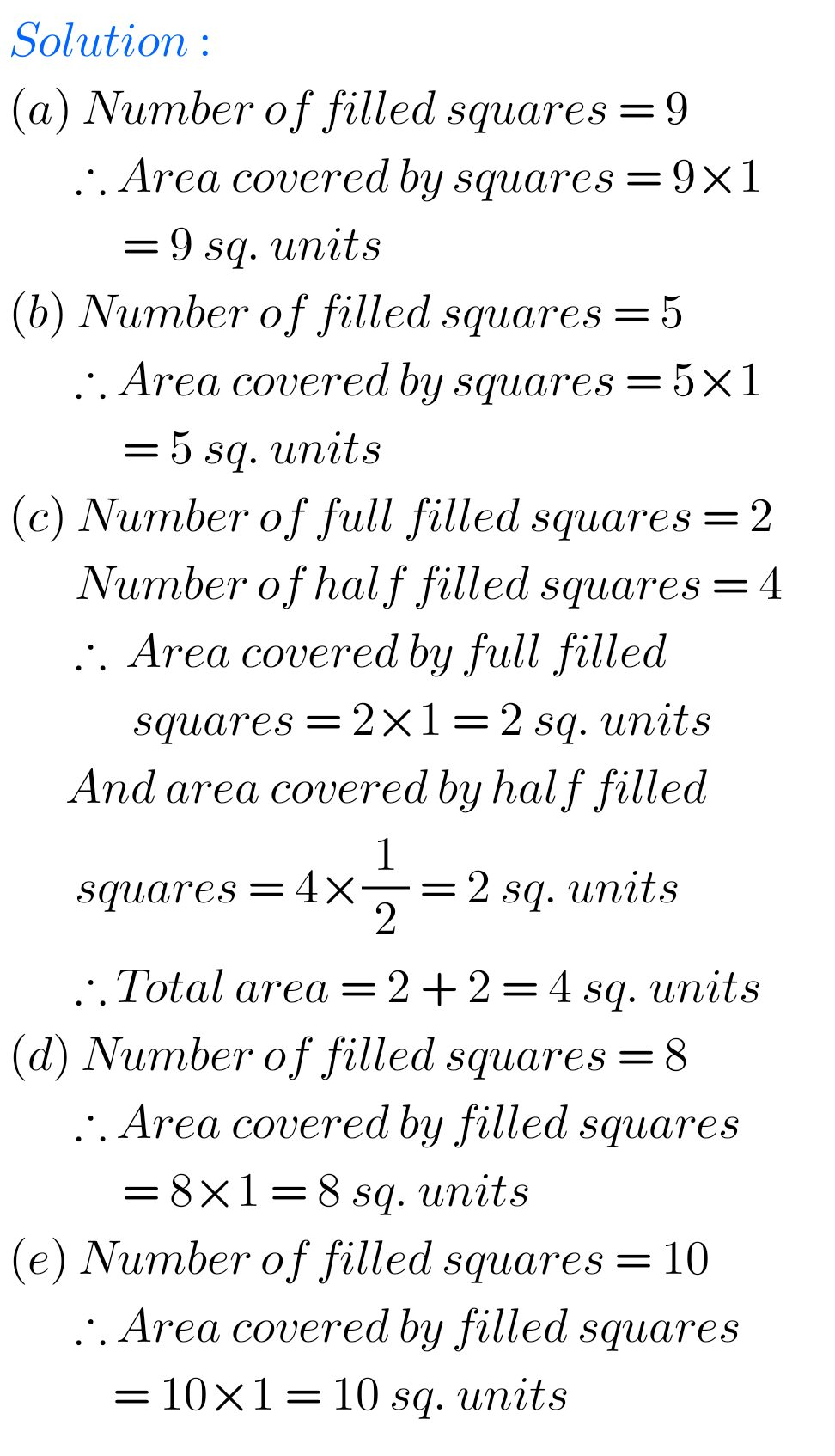# Maths solutions for Mensuration Ncert class 6## Ncert solutions for Mensuration chapter 10 exercise 10.3 class 6

Class 6 chapter 10 Mensuration exercise 10.3 solutions Class 6 Maths Ncert Chapter 10 Mensuration Exercise 10.3 solutions are given. Study the tectbook lesson Mensiration very well. Observe the example problems and solutions given in the tectbook. Observe the given solutions and try them in your own method. You can see Ncert maths solutions for …## Mensuration Exercise 10.2 solutions chapter 10 class 6 ncert maths

Ncert solutions class 6 maths exercise 10.2 Mensuration Class 6 Maths Ncert Chapter 10 Mensuration Exercise 10.2 solutions are given. Study the tectbook lesson Mensiration very well. Observe the example problems and solutions given in the tectbook. Observe the given solutions and try them in your own method. You can see Ncert maths solutions for …## Ncert Maths solutions for Mensuration chapter 10 exercise 10.1 class 6

Mensuration solutions class 6 maths exercise 10.1 Class 6 Maths Ncert Chapter 10 Mensuration Exercise 10.1 solutions are given. Study the tectbook lesson Mensiration very well. Observe the example problems and solutions given in the tectbook. Observe the given solutions and try them in your own method. You can see Ncert maths solutions for class …